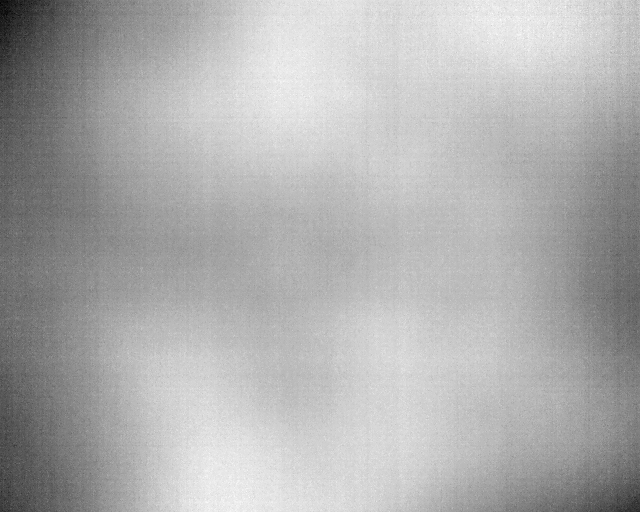Nearly black QPixmap from QImage when using a converted 16-bit image

• I have the following code which works perfectly well on 8-bit grayscale images (OpenCV for loading):

auto display_image = image.clone();

QImage::Format format = QImage::Format_Grayscale8;

QImage qi(display_image.data, display_image.cols, display_image.rows, format);
qi.save("temp_qimage.png");

pixmap.fromImage(qi);
pixmap.save("temp_pixmap.png");

// Do other stuff to display the pixmap etc.

I've added the calls to save for debugging. I then tried to do the same for a 16-bit image, scaled to 8-bit.:

if(image.elemSize() == 2){
// Basic 16-bit crush
double minval, maxval;
cv::minMaxIdx(image, &minval, &maxval);
double range = maxval-minval;
double scale_factor = 255.0/range;

display_image -= minval;
display_image *= scale_factor;
display_image.convertTo(display_image, CV_8U);
}

The resulting QImage is still saved correctly, but the pixmap is now uniform dark grey (everything gets set to an intensity of 29 - not sure if it's different for every picture). I also tried saving with OpenCV and running a histogram, the conversion is definitely an 8-bit image.

Is there something missing here? As far as I can tell there's no difference between me loading an 8-bit image vs a 16-bit image and converting.

Image:Pixmap:(The raw is a tiff file, which the forum won't let me upload).

EDIT: I've had a play and it seems that if I generate a QImage manually, converting it to a QPixmap fails. For example:

QImage MainWindow::convert16(const cv::Mat &source){
short* pSource = reinterpret_cast<short*>(source.data);

QImage dest(512, 640, QImage::Format_RGB32);
int pixelCounts = dest.width() * dest.height();

double minval, maxval;
cv::minMaxIdx(source, &minval, &maxval);
double range = maxval-minval;
double scale_factor = 255.0/range;

uchar* pDest = dest.bits();

for (int i = 0; i < pixelCounts; i++)
{
uchar value = 255; //(uchar) (*(pSource) - minval)*(255.0/range);
*(pDest++) = value;  // B
*(pDest++) = 0;  // G
*(pDest++) = 0;  // R
*(pDest++) = 0;      // Alpha
pSource++;
}

dest.save("test.png");

return dest;
}

the code has been modified to produce a completely blue image, but the pixmap (e.g. pixmap.fromImage(convert16())) is still black. On the other hand, this works:

QTemporaryDir dir;
if (dir.isValid()) {
convert16(display_image).save(dir.path()+"/temp.png");